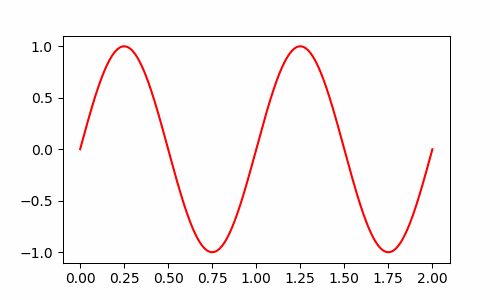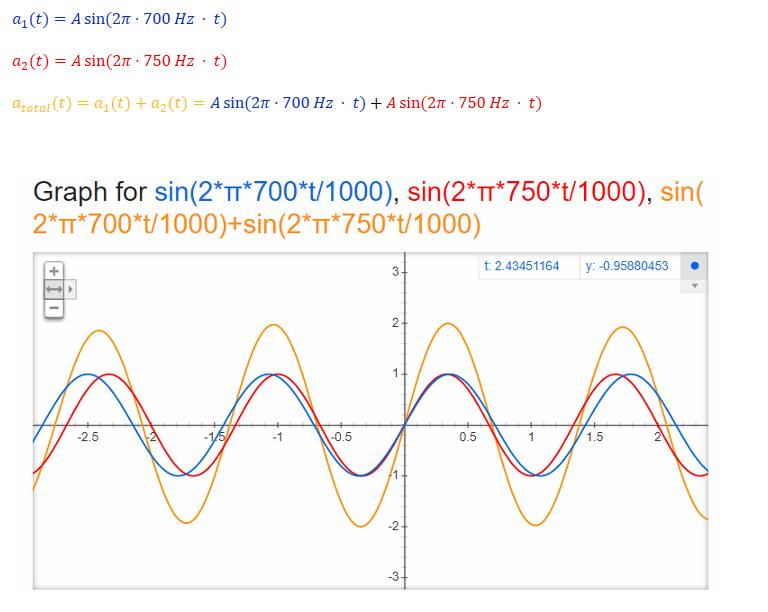top of page
Search

# Waves, sound and Doppler effect tutorialQUESTION 1: "The end of a rope is moved up and down once per second causing waves to travel down its length. Determine the 'amplitude', 'wavelength' and 'frequency' from the attached picture and write down an equation that describes the wave."Let's make it clear what is meant by the terms:If we look at the picture, we can see that the bumps and dips are all 0.2m away from the middle of the rope. Therefore the "amplitude" is A=0.2m The distance between two bumps is 0.5m, which tells us that the wavelength is L=0.5m. We know that the rope is shaken up and down every 0.6 seconds. We say that its "Period" is 0.6s. The number of times per second it gets shaken up and down will be f=1/0.6s = 1.67 Hz (Hertz). Ok, how do we write this as an equation? Here is the general form:Here is how to think about this equation: "How much the ropes is pulled up or down depends on the 'Amplitude' multiplied by the sine of two terms. The first term tells you how often one point of the rope goes up and down when you let time increase. If you take a snapshot of the rope, then the second term tells you how the rope goes up and down as you move along its length." Let's plug in the values we found:If we actually jiggle the rope up and down, we can see that each peak moves forward with a certain speed. It turns out that the speed of a wave can be calculated using the frequency and the wavelength:So a bump on the rope moves forward with as peed of 0.835 meters every second. QUESTION 2: "The siren from an ambulance driving at 30 m/s emits a loud, clear tone in order to alert cars in traffic. The driver of the ambulance hears this tone as having a frequency of 700Hz. What frequency is heard by a pedestrian standing in front of the ambulance? What about a pedestrian standing behind it?" Hmm that sounds pretty cryptic. Let's make a drawing of what is going on:Here is the basic idea: A loudspeaker works by pushing on the air molecules in front of it. The siren pushes the air molecules once every 1/700Hz =0.0014 s= 1.4 millisecond. If the siren is mounted on top of a moving vehicle, the following will happen:

1. The siren pushes on the air molecules

2. The "blob" of air molecules moves forward at the speed of sound.

3. The car is moving, so it catches up to the "blob" of air a little bit.

4. The siren pushes on the air again and forms another blob.

Result: The blobs of air "pile up" in front of the ambulance. Someone standing in front of the ambulance will experience a lot of "air blobs" hitting their ear every second. Therefore they hear a higher frequency. Someone standing behind the ambulance will hear a lower frequency because the ambulance moves away from the "blobs" that are emitted in the backwards direction. Basically, the frequency you hear can be calculated from:Just pick + if the ambulance is moving away and - if it is moving towards you. QUESTION 3: "Two loudspeakers are emitting clear tones at 700 Hz and 750 Hz respectively. What sound will you hear if you are standing between them?" Here is how to think about this problem: Where you are standing, there are two devices jiggling the air molecules back and forth. One jiggles them 700 times per second and the other one jiggles them 750 times per second. We can write the two "jiggle functions" down and then add them together:^In the beginning, the two speakers jiggle the air molecules "in synch". Therefore the air molecules get displaced a lot. But as you can see from the graph above, they start to drift out of synch. If we wait a bit longer, they will drift so far that their relative "jiggling" cancel each other out:This effect of jiggling waves "adding together and cancelling each other out" is called "Interference". If we wait a bit longer, the two jiggling effects will get back in synch:What happens if we "zoom out" even further?Weird. The graph seems to say that the air molecules will be jiggled at a high frequency, AND that how much they get jiggled changes with time. We are playing two high frequencies and we end up hearing one high frequency + one low frequency. It will basically sound like the two sounds: "iiiiiiiiiiiiiiiiiiiiii" And "wub wub wub wub" at the same time. We say that these two frequencies generate a "Beat". Let's calculate the frequency of this beat:Use this Trigonometric identity that I have shamelessly copy-pasted from wikipedia:Aha! We see that by adding together the jiggling effects of the two speakers, we get a total jiggling, that is their average jiggling frequency multiplied by their difference.